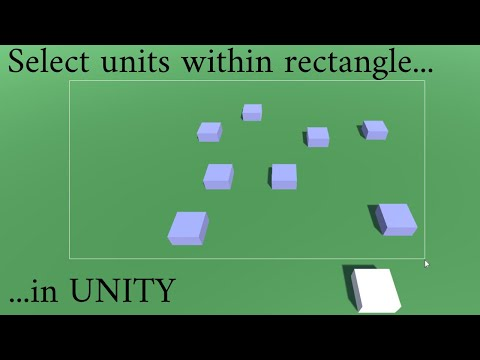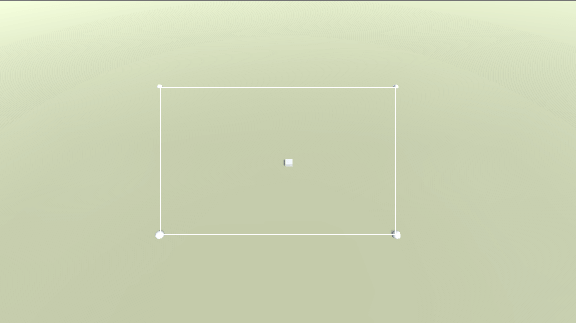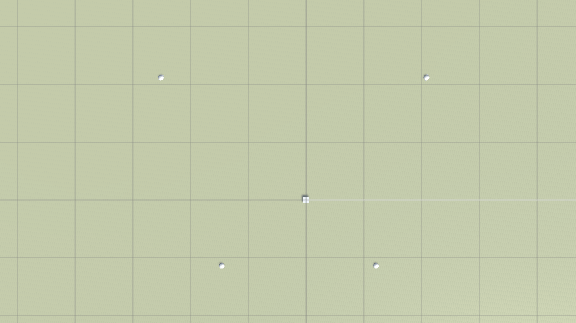# Select units within a rectangle in Unity with C#

In strategy games it's common to select units by making a rectangle with the mouse. All of those units within the area will then be selected when you release the mouse button. This is how it looks like in the strategy game Red Alert:I've recently been prototyping a strategy game and I realized that selecting all units within a rectangle wasn't as easy as I first had thought. The difference with the classic strategy games is that we are now operating in 3D space and not 2D space as in Red Alert. So we will se that we are not selecting units within a rectangle in 3D space, but the corners in the rectangle are actually not 90 degrees, even though it looks like the corners are 90 degrees on the screen.

The result will hopefully look something like this YouTube video:### Basic scene

But before we begin with the heavy lifting we need a basic scene. So just create a ground plane (make sure it's on layer 8 or change it in the code) and a few cubes that will act as our units (make sure they are taged as Friendly or change it in the code). A unit can either be:

• Selected. We have selected the unit (and in a real game we can now move the unit).
• Not selected. The unit is just standing there.
• Highlighted. This mode is useful if we just want to display the unit's health (like in Red Alert). We are either hovering with the mouse above the unit or the unit is within the selection rectangle but we haven't released the mouse button so the unit is not selected.

So we need 3 different materials to show if a unit is either highlighted, selected, or not anything at all. Also add 4 spheres to make it easier to debug the corners of the rectangle in which we will select the units.

You also need a camera script. A basic script that will do the job:

```using UnityEngine;
using System.Collections;

namespace Tutorial
{
public class TiltedCamera : MonoBehaviour
{
//The height we begin with
public float startZoom;

//The camera's transform to save space
Transform cameraTrans;

void Start()
{
//Get the camera's transform
cameraTrans = Camera.main.transform;

//Move the camera to the start position
cameraTrans.position = Vector3.zero;

//Rotate the camera to the correct rotation
cameraTrans.eulerAngles = new Vector3(45f, 0f, 0f);

//Zoom the camera to the initial zoom
cameraTrans.Translate(-Vector3.forward * startZoom);
}
}
}
```

### Make the square

When I first began trying to make a rectangle I though about making a line renderer, but it didn't look good. So we are instead going to use Unity's GUI system. So add an "UI Image" to the scene. This will automatically add a canvas and the Image will be a child to the canvas. Rename the Image to Selection Square.

The square itself will consist of a tiny 3x3 pixels large image, where the center is transparent and the surrounding frame is the color you want the selection rectangle to consist of. Click here to download it.

Change it to Texture type: Sprite (2D and UI), and add the 3x3 image as source image in the GUI Image object. Now in the GUI Image object you change the Image type to Sliced, but now Unity will complain that "This image doesn't have any borders."To give it borders, select the image and click on Sprite editor. From the different sides of the image, drag to form borders across the center pixel:Then click on apply and try to resize the GUI Image object (and uncheck Fill Center). You should now see that the border is always 1 pixel large no matter how large the image is. Also make sure the transform is anchored to the middle of the canvas.### Draw the square

The idea here is that we are going to:

• Select a single unit will Left Mouse Button
• Select all units within a rectangle by making a square while holding Left Mouse Button
• Deselect all units by clicking with Left Mouse Button on something else than a unit
• Highlight a unit while hovering above with the mouse, or if the unit is inside of the rectangle

To make all this work we need to determine if we are clicking with the left mouse button or holding it to make a rectangle. We are going to do that by using a timer. So if we have held down the mouse button for a certain amount of time, we will assume that we are going to make a rectangle. Otherwise we assume that we are clicking with the mouse button. But that's the easy part.

The complicated part is to determine the corners of the rectangle, and then draw the GUI rectangle, and then check if a unit is within the rectangle. To do that we have to convert the world position where we started the rectangle to a GUI position, which is in 2D. When we have done that we figure out the center position of the rectangle we want to draw. And if we have the start position and end position (like top-left and bottom-right corner) we can easily calculate the width and the height of the rectangle. We then add the center position and the size to the GUI rectangle image we created.

When we know the size of the GUI rectangle, we have to convert the corners back to the 3D world because two of the corners are not the same as if we had made the same calculations in 3D space. If you test to make a rectangle, then pause the game, and look at the scene from the top, you will see that what looks like a rectangle in 2D is actually not a rectangle in 3D.So to determine if a unit is within the polygon we are going to divide it into two triangles and then just check if the center position of a unit is within one of the triangles. If you want you could check all corners of your unit, but it will be a little slower.

Anyway, this is the final script:

```using UnityEngine;
using System.Collections;
using System.Collections.Generic;

namespace Tutorial
{
public class SelectionSquare : MonoBehaviour
{
//Add all units in the scene to this array
public GameObject[] allUnits;
//The selection square we draw when we drag the mouse to select units
public RectTransform selectionSquareTrans;
//To test the square's corners
public Transform sphere1;
public Transform sphere2;
public Transform sphere3;
public Transform sphere4;
//The materials
public Material normalMaterial;
public Material highlightMaterial;
public Material selectedMaterial;

//All currently selected units
[System.NonSerialized]
public List<GameObject> selectedUnits = new List<GameObject>();

//We have hovered above this unit, so we can deselect it next update
//and dont have to loop through all units
GameObject highlightThisUnit;

//To determine if we are clicking with left mouse or holding down left mouse
float delay = 0.3f;
float clickTime = 0f;
//The start and end coordinates of the square we are making
Vector3 squareStartPos;
Vector3 squareEndPos;
//If it was possible to create a square
bool hasCreatedSquare;
//The selection squares 4 corner positions
Vector3 TL, TR, BL, BR;

void Start()
{
//Deactivate the square selection image
selectionSquareTrans.gameObject.SetActive(false);
}

void Update()
{
//Select one or several units by clicking or draging the mouse
SelectUnits();

//Highlight by hovering with mouse above a unit which is not selected
HighlightUnit();
}

//Select units with click or by draging the mouse
void SelectUnits()
{
//Are we clicking with left mouse or holding down left mouse
bool isClicking = false;
bool isHoldingDown = false;

//Click the mouse button
if (Input.GetMouseButtonDown(0))
{
clickTime = Time.time;

//We dont yet know if we are drawing a square, but we need the first coordinate in case we do draw a square
RaycastHit hit;
//Fire ray from camera
if (Physics.Raycast(Camera.main.ScreenPointToRay(Input.mousePosition), out hit, 200f, 1 << 8))
{
//The corner position of the square
squareStartPos = hit.point;
}
}
//Release the mouse button
if (Input.GetMouseButtonUp(0))
{
if (Time.time - clickTime <= delay)
{
isClicking = true;
}

//Select all units within the square if we have created a square
if (hasCreatedSquare)
{
hasCreatedSquare = false;

//Deactivate the square selection image
selectionSquareTrans.gameObject.SetActive(false);

//Clear the list with selected unit
selectedUnits.Clear();

//Select the units
for (int i = 0; i < allUnits.Length; i++)
{
GameObject currentUnit = allUnits[i];

//Is this unit within the square
if (IsWithinPolygon(currentUnit.transform.position))
{
currentUnit.GetComponent<MeshRenderer>().material = selectedMaterial;

}
//Otherwise deselect the unit if it's not in the square
else
{
currentUnit.GetComponent<MeshRenderer>().material = normalMaterial;
}
}
}

}
//Holding down the mouse button
if (Input.GetMouseButton(0))
{
if (Time.time - clickTime > delay)
{
isHoldingDown = true;
}
}

//Select one unit with left mouse and deselect all units with left mouse by clicking on what's not a unit
if (isClicking)
{
//Deselect all units
for (int i = 0; i < selectedUnits.Count; i++)
{
selectedUnits[i].GetComponent<MeshRenderer>().material = normalMaterial;
}

//Clear the list with selected units
selectedUnits.Clear();

//Try to select a new unit
RaycastHit hit;
//Fire ray from camera
if (Physics.Raycast(Camera.main.ScreenPointToRay(Input.mousePosition), out hit, 200f))
{
//Did we hit a friendly unit?
if (hit.collider.CompareTag("Friendly"))
{
GameObject activeUnit = hit.collider.gameObject;
//Set this unit to selected
activeUnit.GetComponent<MeshRenderer>().material = selectedMaterial;
//Add it to the list of selected units, which is now just 1 unit
}
}
}

//Drag the mouse to select all units within the square
if (isHoldingDown)
{
//Activate the square selection image
if (!selectionSquareTrans.gameObject.activeInHierarchy)
{
selectionSquareTrans.gameObject.SetActive(true);
}

//Get the latest coordinate of the square
squareEndPos = Input.mousePosition;

//Display the selection with a GUI image
DisplaySquare();

//Highlight the units within the selection square, but don't select the units
if (hasCreatedSquare)
{
for (int i = 0; i < allUnits.Length; i++)
{
GameObject currentUnit = allUnits[i];

//Is this unit within the square
if (IsWithinPolygon(currentUnit.transform.position))
{
currentUnit.GetComponent<MeshRenderer>().material = highlightMaterial;
}
//Otherwise deactivate
else
{
currentUnit.GetComponent<MeshRenderer>().material = normalMaterial;
}
}
}
}
}

//Highlight a unit when mouse is above it
void HighlightUnit()
{
//Change material on the latest unit we highlighted
if (highlightThisUnit != null)
{
//But make sure the unit we want to change material on is not selected
bool isSelected = false;
for (int i = 0; i < selectedUnits.Count; i++)
{
if (selectedUnits[i] == highlightThisUnit)
{
isSelected = true;
break;
}
}

if (!isSelected)
{
highlightThisUnit.GetComponent<MeshRenderer>().material = normalMaterial;
}

highlightThisUnit = null;
}

//Fire a ray from the mouse position to get the unit we want to highlight
RaycastHit hit;
//Fire ray from camera
if (Physics.Raycast(Camera.main.ScreenPointToRay(Input.mousePosition), out hit, 200f))
{
//Did we hit a friendly unit?
if (hit.collider.CompareTag("Friendly"))
{
//Get the object we hit
GameObject currentObj = hit.collider.gameObject;

//Highlight this unit if it's not selected
bool isSelected = false;
for (int i = 0; i < selectedUnits.Count; i++)
{
if (selectedUnits[i] == currentObj)
{
isSelected = true;
break;
}
}

if (!isSelected)
{
highlightThisUnit = currentObj;

highlightThisUnit.GetComponent<MeshRenderer>().material = highlightMaterial;
}
}
}
}

//Is a unit within a polygon determined by 4 corners
bool IsWithinPolygon(Vector3 unitPos)
{
bool isWithinPolygon = false;

//The polygon forms 2 triangles, so we need to check if a point is within any of the triangles
//Triangle 1: TL - BL - TR
if (IsWithinTriangle(unitPos, TL, BL, TR))
{
return true;
}

//Triangle 2: TR - BL - BR
if (IsWithinTriangle(unitPos, TR, BL, BR))
{
return true;
}

return isWithinPolygon;
}

//Is a point within a triangle
//From http://totologic.blogspot.se/2014/01/accurate-point-in-triangle-test.html
bool IsWithinTriangle(Vector3 p, Vector3 p1, Vector3 p2, Vector3 p3)
{
bool isWithinTriangle = false;

//Need to set z -> y because of other coordinate system
float denominator = ((p2.z - p3.z) * (p1.x - p3.x) + (p3.x - p2.x) * (p1.z - p3.z));

float a = ((p2.z - p3.z) * (p.x - p3.x) + (p3.x - p2.x) * (p.z - p3.z)) / denominator;
float b = ((p3.z - p1.z) * (p.x - p3.x) + (p1.x - p3.x) * (p.z - p3.z)) / denominator;
float c = 1 - a - b;

//The point is within the triangle if 0 <= a <= 1 and 0 <= b <= 1 and 0 <= c <= 1
if (a >= 0f && a <= 1f && b >= 0f && b <= 1f && c >= 0f && c <= 1f)
{
isWithinTriangle = true;
}

return isWithinTriangle;
}

//Display the selection with a GUI square
void DisplaySquare()
{
//The start position of the square is in 3d space, or the first coordinate will move
//as we move the camera which is not what we want
Vector3 squareStartScreen = Camera.main.WorldToScreenPoint(squareStartPos);

squareStartScreen.z = 0f;

//Get the middle position of the square
Vector3 middle = (squareStartScreen + squareEndPos) / 2f;

//Set the middle position of the GUI square
selectionSquareTrans.position = middle;

//Change the size of the square
float sizeX = Mathf.Abs(squareStartScreen.x - squareEndPos.x);
float sizeY = Mathf.Abs(squareStartScreen.y - squareEndPos.y);

//Set the size of the square
selectionSquareTrans.sizeDelta = new Vector2(sizeX, sizeY);

//The problem is that the corners in the 2d square is not the same as in 3d space
//To get corners, we have to fire a ray from the screen
//We have 2 of the corner positions, but we don't know which,
//so we can figure it out or fire 4 raycasts
TL = new Vector3(middle.x - sizeX / 2f, middle.y + sizeY / 2f, 0f);
TR = new Vector3(middle.x + sizeX / 2f, middle.y + sizeY / 2f, 0f);
BL = new Vector3(middle.x - sizeX / 2f, middle.y - sizeY / 2f, 0f);
BR = new Vector3(middle.x + sizeX / 2f, middle.y - sizeY / 2f, 0f);

//From screen to world
RaycastHit hit;
int i = 0;
//Fire ray from camera
if (Physics.Raycast(Camera.main.ScreenPointToRay(TL), out hit, 200f, 1 << 8))
{
TL = hit.point;
i++;
}
if (Physics.Raycast(Camera.main.ScreenPointToRay(TR), out hit, 200f, 1 << 8))
{
TR = hit.point;
i++;
}
if (Physics.Raycast(Camera.main.ScreenPointToRay(BL), out hit, 200f, 1 << 8))
{
BL = hit.point;
i++;
}
if (Physics.Raycast(Camera.main.ScreenPointToRay(BR), out hit, 200f, 1 << 8))
{
BR = hit.point;
i++;
}

//Could we create a square?
hasCreatedSquare = false;

//We could find 4 points
if (i == 4)
{
//Display the corners for debug
//sphere1.position = TL;
//sphere2.position = TR;
//sphere3.position = BL;
//sphere4.position = BR;

hasCreatedSquare = true;
}
}
}
}
```

That's it! What you have should now look like this:If you prefer an audio version of this tutorial, this guy is using this tutorial to make a strategy game: Unity Tutorial - RTS Controls - Selection Box GUI - Part 4